Electricity and Magnetism

# Circuit Behavior: Level 4-5 Challenges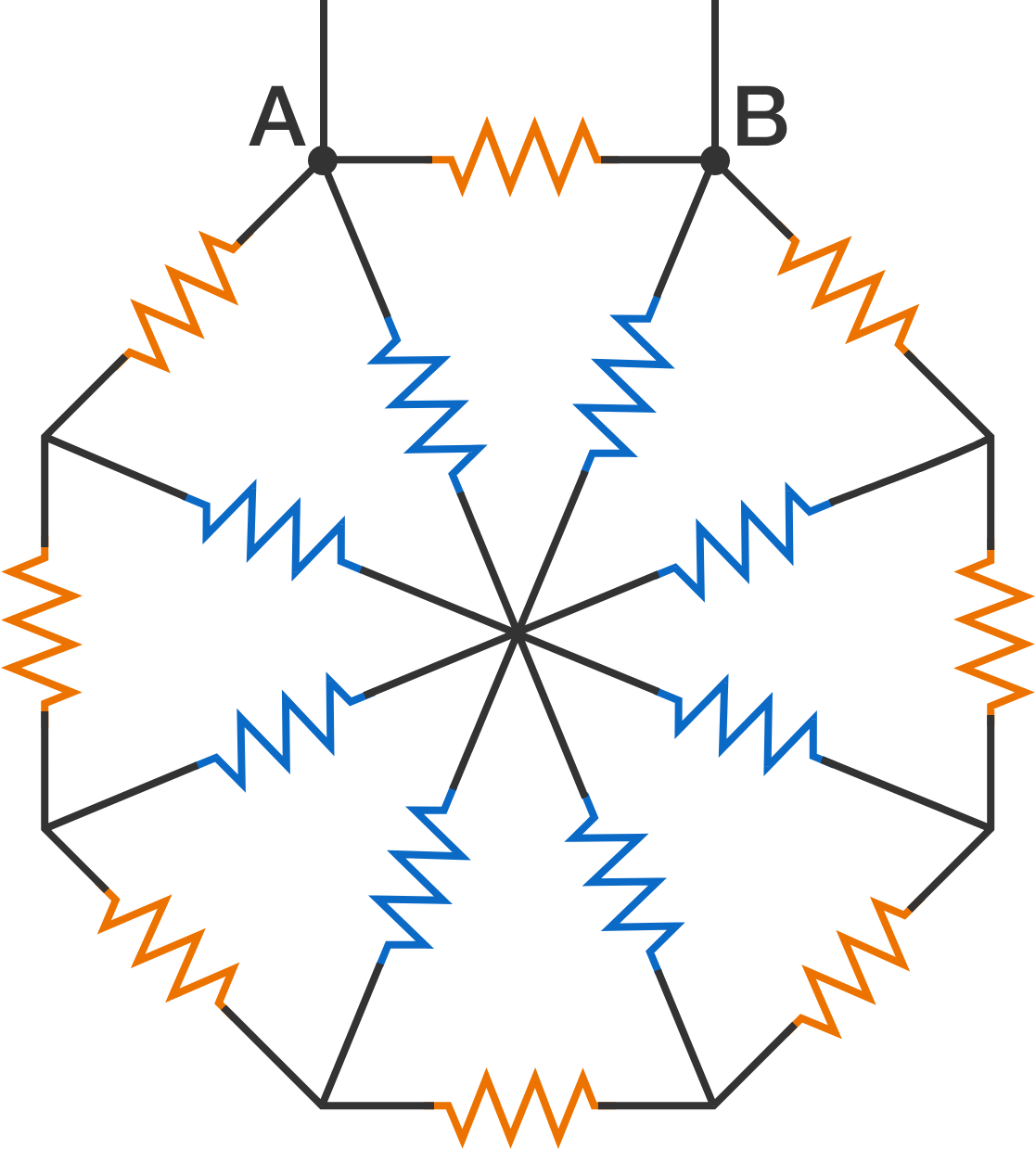8 resistors (orange color) are connected to form a regular octagon. 8 more resistors (blue color) connect the vertices of the octagon to its center. All the 16 resistors are of resistance $\SI{420}{\ohm}$.

If the connecting wires have negligible resistance, calculate the equivalent resistance (in ohms, rounded to the nearest integer) between the terminals $A$ and $B$.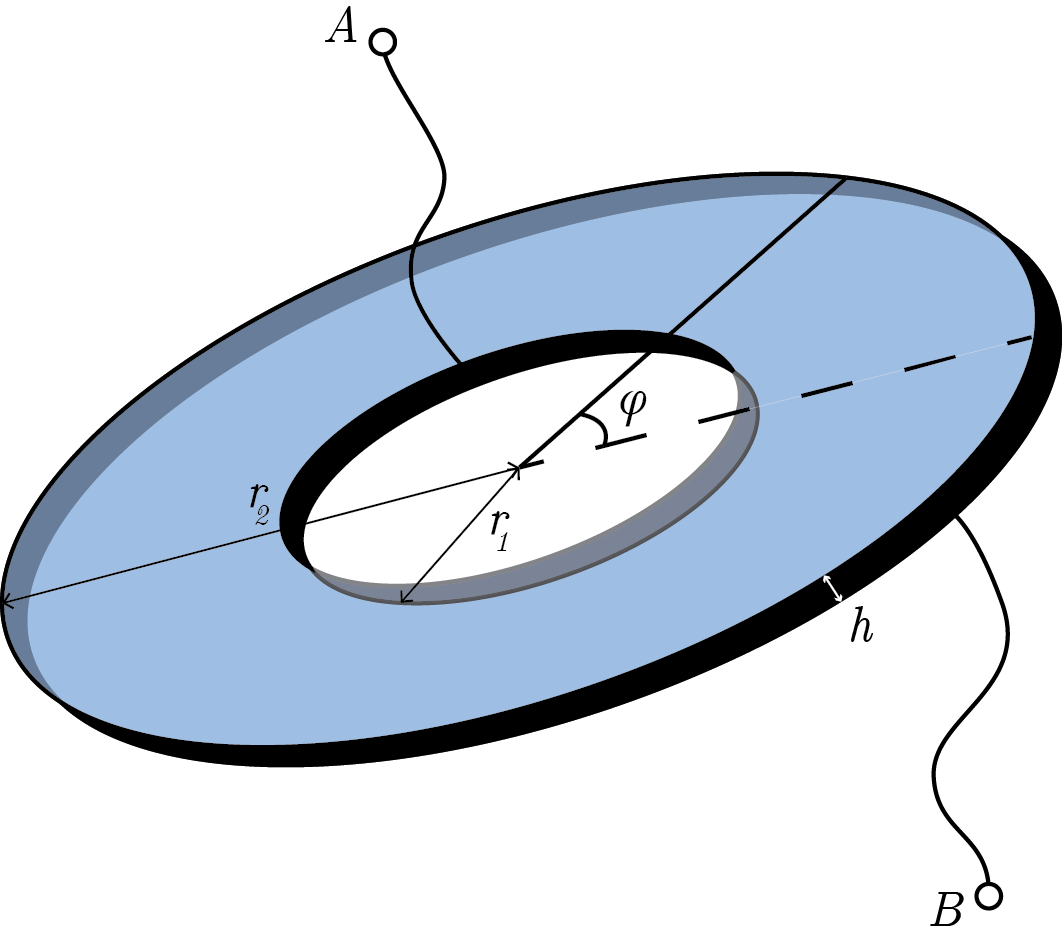You are given a disk of thickness $h$ with inner and outer radii $r_1$ and $r_2$, respectively. If the resistivity of the disk varies as $\rho = \rho_0 \left|\sec \varphi\right|$, where $\varphi$ is the polar angle, find the resistance between the points $A$ and $B$.

Details and Assumptions:

• The inner and outer rims are metal rings with zero resistance.
• Take $\dfrac {r_2}{r_1} = e^2 \approx 7.389$, $\rho_0 = \SI{10}{\ohm \meter}$, and $h= \SI{3}{\centi \meter}$.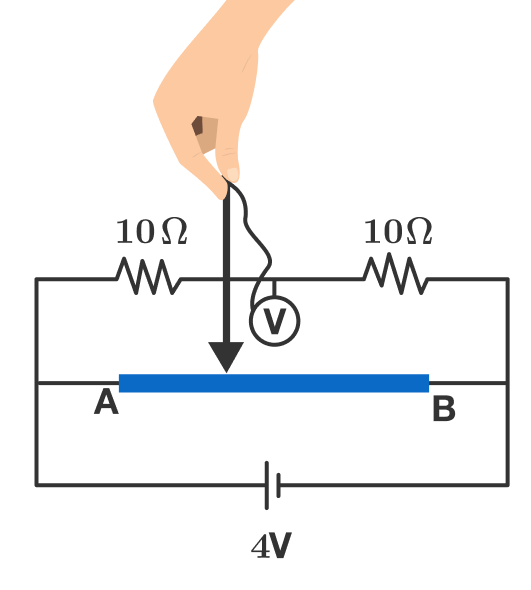In the circuit above, wire $AB$ has length $40\text{ cm}$ and resistance per unit length $\SI[per-mode=symbol]{0.5}{\ohm\per\centi\meter}$. The voltmeter is ideal.

If we want to make the reading in the voltmeter vary with time as $V(t) = 2 \sin(\pi t) \ \si{\volt},$ then what should be the velocity of the contact (the arrow-tipped end of the wire above) as a function of time?

If the velocity can be expressed as $A\sin(\omega t+\phi) \text{ cm}\,\text{s}^{-1},$ where $0<\phi<\pi$, then enter the value of $\dfrac A{\omega- \phi}$.

A useless wire having a total resistance of $48 \space \Omega$ is cut into 48 equal pieces. Then, a regular Deltoidal Icositetrahedron as shown below.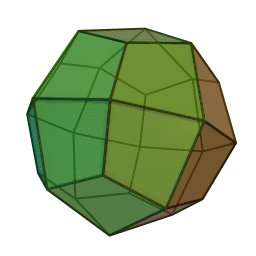If the equivalent resistance between two opposite points, where four edges meet together is $R \space \Omega$, then enter your answer as the value of $100R$.

###### Image credit: Wikipedia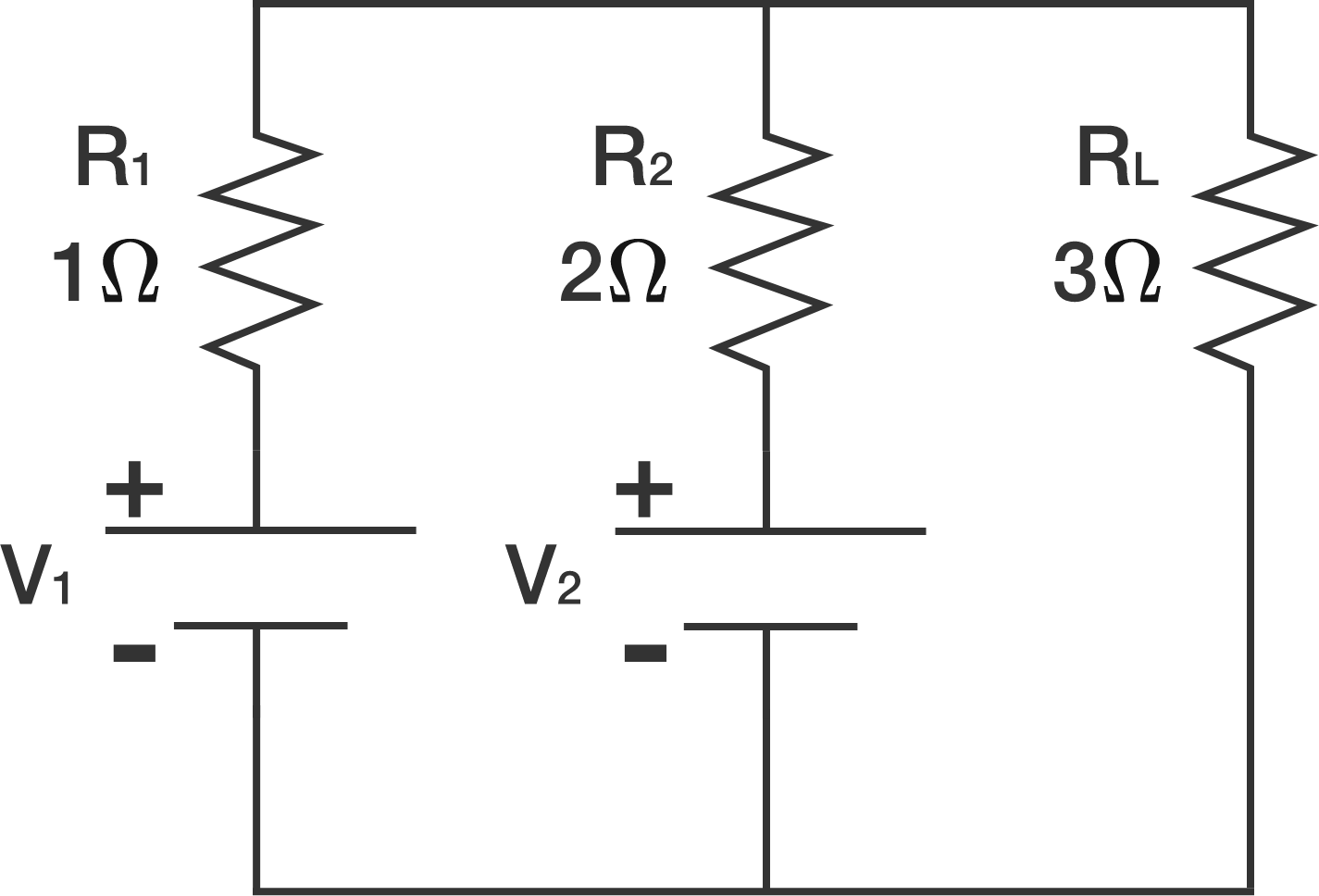The DC circuit above consists of two voltage sources $V_1$ and $V_2$ with internal resistances $R_1 = 1 \Omega$ and $R_2 = 2 \Omega$ respectively. There is a load $R_L = 3 \Omega$ connected in parallel with the sources.

$V_1$ and $V_2$ are variable quantities.

Let $P_1$, $P_2$, and $P_L$ be the amounts of power in watts dissipated by $R_1$, $R_2$, and $R_L$ respectively.

Given that $P_L = 10$ watts, determine the minimum possible value of $P_1 + P_2 + P_L$ to 1 decimal place.

×